# pointers parameters and const

Note: All Examples are written in C++

# Pointers

Pointers are "variables" that do not hold actual content but the address where the content can be found.

We first should make sure that we know the underlying technologie. Whenever we declare a variable/object, this requires some memory in which the content is stored. We can think of the memory as a table, we store our content in the rows of the table where each row has a unique address.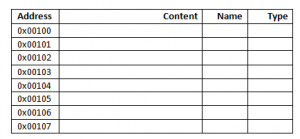So lets assume that we now declare a variable

Then (usually) the Operating system decides where to store this variable. Lets assume it is stored at 0x00101 then our memory would look like this: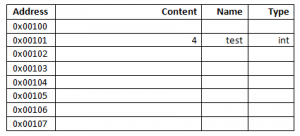whenever we now use the variable, it is known where to find the corresponding value.

We need to introduce 2 operators:

##### Reference operator (&)

The reference operator is giving us back the address of a variable.

would therefore give us 0x00101 as this is the address of "test".

##### Dereference operator (*)

The dereference operator works the other direction of the reference operator. If we give have stored an address then we can use the dereference operator to access the content. That is the basic mechanism of pointers.

Lets assume we want to declare a pointer to an integer. We use an asterisk (*) to tell the compiler that this is not an integer but a pointer to an integer:

Its good coding practice to name the pointers with the prefix "p_" (or similar) so its clear to everyone that this is a pointer. Of course this pointer will also require some memory by itself as the address it is holding need to be saved somewhere.

Right now there is no content, the only thing we did so far is that we declared the pointer, no integer has been declared so far! Our memory could like like this: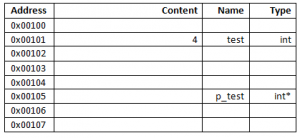We now want to make our pointer to point to the integer "test" we have defined before:

which means that we get the adress of "test" (0x00101) and save it to "p_test".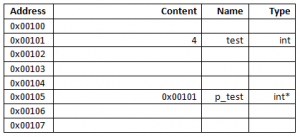We now have a complete pointer. Its obvious that if we access p_test like a regular variable, we get back the the address (the Content) of it but not the value of the integer we pointed to.

is giving us 0x00101.

If we want to access the content of the value we are pointing to we now need to use the dereference operator:

is giving us "4" as we look up the content at 0x00101.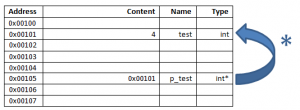Also we can use the dereference operator to get the address of "p_test"

is giving us 0x00105 as this is the address where the pointer is stored.

From the memory above we can now create the following tables: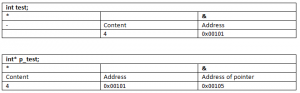##### Pointers to pointers

We can use pointers to other pointers:

So first we dereference "pointer_to_integer_pointer" which is giving us the content of "pointer_to_integer" which is the address to "normalinteger", so we dereference this address again to get the actual value.

##### "new" and "delete"

So far we always have declared variables which are directly coupled to a variable name, like
int myint=1337; .

There is also the possibility to instantiate variables/objects which are not coupled to a variable name. All we get is an address in the memory. We then can use a pointer to access this variable/object. We do so by using the "new" keyword.

It is very important to delete this variable/object after we have used it to make sure the memory is freed so it can be used otherwise.

delete *p_to_int;

Please note that the address of the deleted object is still stored within the pointer and it might be possible to still use it. BUT the memory is not allocated to us anymore, so it can change at any time which can lead to big catastrophes. Therefore very often the pointer is assigned to "null" to indicate that it is not in use at the moment. However, the memory allocated is freed if the program ends, therefore its up to you to decide whether its usefull to free the memory.

##### Pointers and Arrays

Pointers can be used to control arrays. After we have declared an array, we can use a pointer to point to the first address of the array. We then can use increment and decrement operators to navigate through the array.

As we have seen above we can use pointers to point to elements within an array. Also the array can be filled with pointers:

The memory in the example could look like this if we place "p_pointer_to_integer" to the first element in the array.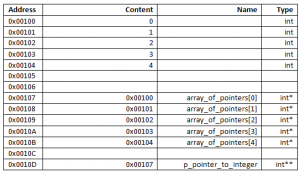# parameter passing

There are different ways to pass parameters to a function/method.

### Call by value

If we pass parameters by call by value we copy the parameter to a inner variable, this has the benefit that the variable which is used within the function/method is an own copy of the parameter, on the other hand we can not use this variable to pass content out of the function/method and also we need more memory to realize this.

In the example above the values of "m_var1" and "m_var2" are copied to "var1" and "var2".
"var1" and "var2" then can be used inside "call_by_value".

### Call by pointer

If we call by pointer then we will give the address(es) where the parameters can be found to the function/method. We now can write directly to the given parameters (to m_var1,m_var2) from within the function/method. This has the benefit that we do not occupy additional memory and do not need to copy the values, but on the other hand, if there is something going wrong within the function/method, the original values might be lost. Note that we first need to dereference the pointer to access its content.

### Call by reference

This approach is very similar to call by pointer but has one difference, we do not need to dereference when assigning a value and we do not need to get the address when calling the function/method.

The problem here is that it is not obvious at the first sight that we are dealing with a reference and not with an own value. To avoid misunderstandings we can use "const".

# Const

"Const" can be used to disallow a value to be overwritten.
For normal variables its quite easy:

const double pi=3.1415926535897932384626433832795028841971693993751058209749445923078164;

will disallow to overwrite "pi" with another value.

For Parameter Passing with call by reference or pointer its a bit more complicated. Here it depends on the position. Is it left/right of */& or on both sides.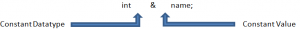If the Datatype is constant the dereferenced value can not be changed.
If the Value (aka the Address) is constant, it can not be changed, so we can not point to another instance.

If you use "const" for the return value of a function/method then the return value is changeable in its content but not in its address.